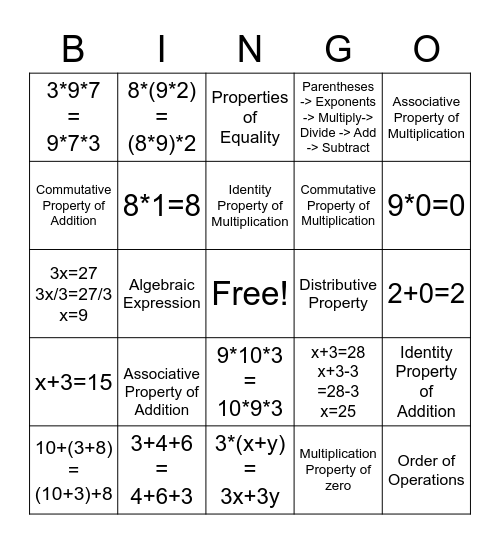# Properties of Numbers BingoThis bingo card has a free space and 24 words: Associative Property of Addition, 3+4+6 = 4+6+3, Identity Property of Multiplication, 10+(3+8) =(10+3)+8, Associative Property of Multiplication, 3*9*7 = 9*7*3, Commutative Property of Addition, 8*(9*2) =(8*9)*2, Distributive Property, 9*10*3 = 10*9*3, Commutative Property of Multiplication, 3x=27 3x/3=27/3 x=9, x+3=28 x+3-3 =28-3 x=25, Identity Property of Addition, 8*1=8, Multiplication Property of zero, 2+0=2, Properties of Equality, 9*0=0, Algebraic Expression, Order of Operations, x+3=15, Parentheses -> Exponents -> Multiply-> Divide -> Add -> Subtract and 3*(x+y) = 3x+3y.

## Play Online

Share this URL with your players:For more control of your online game, create a clone of this card first.

## Probabilities

With players vying for a you'll have to call about __ items before someone wins. There's a __% chance that a lucky player would win after calling __ items.

Tip: If you want your game to last longer (on average), add more unique words/images to it.